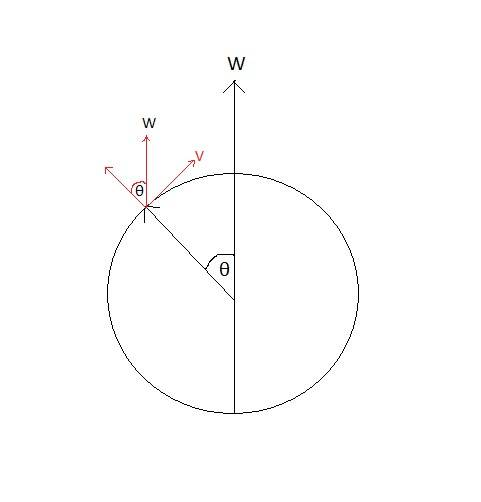# Question about the Coriolis effect

• Foruer
In summary, the deviation of a bullet fired on Earth's surface is 0.041 meters in relation to the target.f

## Homework Statement

It's a question about the deviation of a bullet fired on Earth's surface:
**I solved the question, but I can't figure out what exactly this deviation means, where is it on the following question's picture:The problem:
A bullet is fired from a gun towards a target at distance of D=800 meters.
The bullet speed is V=800 meters per second.
The shot is taken at latitude θ=45°, towards the north:
What is the deviation of the bullet from the target?

ac = -2W×V

## The Attempt at a Solution

Time for bullet to reach target = 1 second (target at 800m, bullet speed is 800 m/s).

W(Earth's rotational speed) = 2π/(24*60*60) = 0.0000727
ac = -2W×V = -2*(0.0000727)*800*sin(45) = 0.082 (m/s2)

And then the deviation is x=at2 / 2 = 0.041 meters.

Where exactly is this deviation?

## Homework Statement

It's a question about the deviation of a bullet fired on Earth's surface:
**I solved the question, but I can't figure out what exactly this deviation means, where is it on the following question's picture:

View attachment 226866
The problem:
A bullet is fired from a gun towards a target at distance of D=800 meters.
The bullet speed is V=800 meters per second.
The shot is taken at latitude θ=45°, towards the north:
What is the deviation of the bullet from the target?

ac = -2W×V

## The Attempt at a Solution

Time for bullet to reach target = 1 second (target at 800m, bullet speed is 800 m/s).

W(Earth's rotational speed) = 2π/(24*60*60) = 0.0000727
ac = -2W×V = -2*(0.0000727)*800*sin(45) = 0.082 (m/s2)

And then the deviation is x=at2 / 2 = 0.041 meters.

Where exactly is this deviation?
The Coriolis force and Coriolis acceleration are vectors, so they have direction, depending on the directions of the angular velocity ##\vec Ω ## and ##\vec v ##, the velocity of the body in the rotating frame of reference . https://hu.wikipedia.org/wiki/Coriolis-erő

•Foruer
Thank you, I do know that, but in this specific problem I can't point to where this 0.041(m) is. I'd like someone to draw a line or any other way to show where exactly is this deviation. it's 0.041(m) in relation to what exactly?

Thank you, I do know that, but in this specific problem I can't point to where this 0.041(m) is. I'd like someone to draw a line or any other way to show where exactly is this deviation. it's 0.041(m) in relation to what exactly?
The Coriolis acceleration is proportional to the vector product of the angular velocity and the velocity of the object. For that you can figure out the direction of the acceleration. Without the Coriolis force, the body would travel in the direction of the target, along the initial velocity. In what direction does it deviate from it because of the Coriolis force?

You can also forget the vectorial product if use spherical coordinates. Then all the accelerations appears in a analitical way from the Chirstoffel Symbols in spherical coordinates.

All signs are referred to the coordinate system. You can see that in the next link, last post (in the link), EXAMPLE 2.
(note that in the link appears all accelerations not only coriolis, but there are explanation for all terms, coriolis also an their sings and direction)

https://www.physicsforums.com/threa...-aceleration-from-christoffel-symbols.923208/

I'm sorry because I can not quote only EXAMPLE 2, and need to post entire link.
Edit: nothe that in the link theta is the angle between the z axis an position vector R.
in your case this are not problem because your latitude is 45º and then sin45º=cos45º.
(note in example 2 that, the cos in equation is equivalent to your sin in vectorial product)
(If you see your own picture the angle between V and W is 90-theta)

Last edited:
Yes, sorry it's 90-theta, but since it's 45 I didn't write it (because it's the same). About the deviation , I still don't know, would like someone to draw on my picture where exactly is it so I can understand :)

Yes, I see that the link is hard to you forget it.

Then we go back to the vectorial product: Ac=-2·WxV
Look in your draw the direction of W and V, and then remember the rule of the thumb of the right hand for the vectorial product. (And the Ac has the oposite direction because the - sign)
And in the link you see Ac is in the ##\varphi## direction... in your case is to the East or to the West...